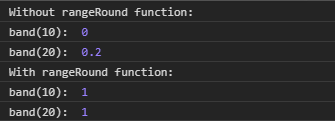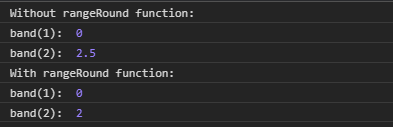Related Articles

# D3.js band.rangeRound() Function

• Last Updated : 24 Aug, 2020

The band.rangeRound() function is used to set the range of the scale to the specified two-element array along with this it also set the round to true.

Syntax:

Hey geek! The constant emerging technologies in the world of web development always keeps the excitement for this subject through the roof. But before you tackle the big projects, we suggest you start by learning the basics. Kickstart your web development journey by learning JS concepts with our JavaScript Course. Now at it's lowest price ever!

`band.rangeRound([range]) `

Parameters: This function accepts single parameters as given above and described below:

• range: This parameter accepts a two-element array of numbers.

Return Values: This function does not return anything.

Below given are a few examples of the function given above.

Example 1:

 ` ``<``html` `lang` `= ``"en"``> ``<``head``> ``    ``<``meta` `charset` `= ``"UTF-8"` `/> ``    ``<``meta` `name` `= ``"viewport"``        ``path1tent = "``width``=``device``-width, ``        ``initial-scale` `= ``1``.0"/> ``    ``<``script` `src` `=``    ``"https://d3js.org/d3.v4.min.js"``>``    ````     ` ` ``<``body``> ``    ``<``script``> ``    ``// Creating the band scale with``    ``// specified domain and range.``        ``var band = d3.scaleBand()``                    ``.domain([10, 20, 30, 40, 50])``                    ``.range([0, 1]);`` ` `        ``console.log("Without rangeRound function:");``        ``console.log("band(10): ", band(10));``        ``console.log("band(20): ", band(20));``       ` `        ``console.log("With rangeRound function:");``       ` `        ``var band = d3.scaleBand()``                    ``.domain([10, 20, 30, 40, 50])``                    ``.rangeRound([0, 1]);``       ` `        ``console.log("band(10): ", band(10));``        ``console.log("band(20): ", band(20));``    `` `` ```

Output:Example 2:

 ` ``<``html` `lang` `= ``"en"``> ``<``head``> ``    ``<``meta` `charset` `= ``"UTF-8"` `/> ``    ``<``meta` `name` `= ``"viewport"``        ``path1tent = "``width``=``device``-width, ``        ``initial-scale` `= ``1``.0"/> ``    ``<``script` `src` `=``    ``"https://d3js.org/d3.v4.min.js"``>``    ````     ` ` ``<``body``> ``    ``<``script``> ``    ``// Creating the band scale with``     ``// specified domain and range.``        ``var band = d3.scaleBand()``                    ``.domain([1, 2, 3, 4])``        ``// When range is in string``                    ``.range(["0", "10"]);``        ``console.log("Without rangeRound function:");``        ``console.log("band(1): ", band(1));``        ``console.log("band(2): ", band(2));``       ` `        ``console.log("With rangeRound function:");``       ` `        ``var band = d3.scaleBand()``                    ``.domain([1, 2, 3, 4, 5])``                    ``.rangeRound(["0", "10"]);``       ` `        ``console.log("band(1): ", band(1));``        ``console.log("band(2): ", band(2));``    `` `` ```

Output:My Personal Notes arrow_drop_up